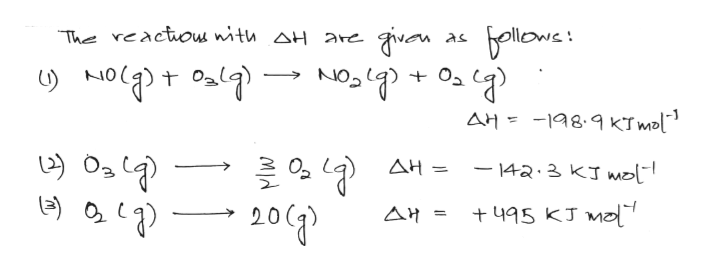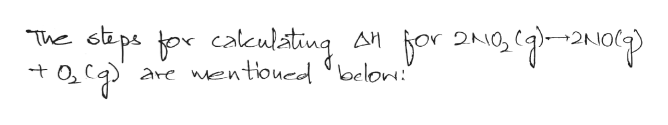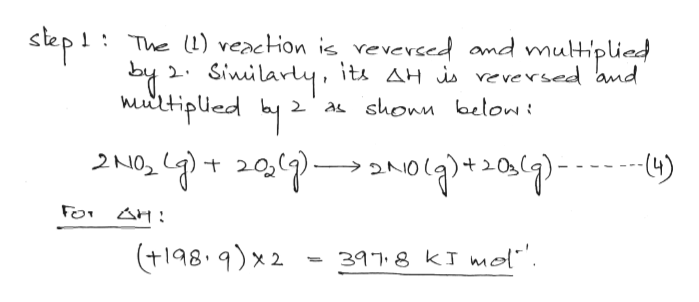Given the following thermochemical equations, NO(g) + O3(g) →NO2(g) + O2(g)ΔH = −198.9 kJ/mol  O3(g) →3/2O2(g) ΔH = −142.3 kJ/mol  O2(g) → 2O(g)ΔH = +495 kJ/mol determine the enthalpy change for the reaction 2NO2(g) →2NO(g) + O2(g) ΔH =  kJ/mol

Question
 Given the following thermochemical equations, NO(g) + O3(g) →NO2(g) + O2(g) ΔH = −198.9 kJ/mol     O3(g) →3/2O2(g)  ΔH = −142.3 kJ/mol     O2(g) → 2O(g) ΔH = +495 kJ/mol  determine the enthalpy change for the reaction 2NO2(g) →2NO(g) + O2(g) ΔH =  kJ/mol
Step 1help_outlineImage Transcriptionclosebellewe The reactou with aH are NO NO t AH= 198.9 KTmol 를요녕 AH 142.3 KJ mo 20 + 495 KJ fullscreen
Step 2help_outlineImage Transcriptionclosesp tor calculating are wentiouedd oelon: The AH fullscreen
Step 3help_outlineImage Transcriptionclose1:The )veaction is veverced and mutiplied 2Sinilarty, its AH i ve versed and 2'a shonm below i 2N02 (4) FO AH (t1989)x2 3978 kJ mol"'. fullscreen

Want to see the full answer?

See Solution

Want to see this answer and more?

Our solutions are written by experts, many with advanced degrees, and available 24/7

See Solution
Tagged in

Physical Chemistry